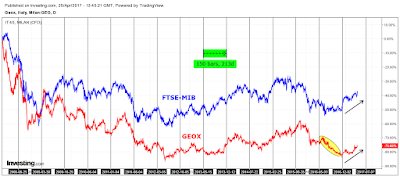## Tuesday, April 25, 2017

### Geox S.p.A. : the historical correlation with the FTSE-mib is significative.

If we look at the historical chart of Geox (red) compared with the FTSE-mib (blue), we can notice that the similitude  is clear : some exceptions apart (like the section of the circled graph, yellow ellipse), the direction of the two assets is substantially the same. To make the comparison more effective, the chart is divided into vertical lines that represent the date ranges with equal period (150 bars) : the most important thing is to value the same direction of the two graphs, inside the date range, in order to have the correlation ; otherwise, the two assets would be unrelated.
In this way, the last performance of Geox is driven by the trend of the FTSE-mib, most likely.
Indeed, the positive newsflow is absent and the fundamentals are weak (see the last news) : they don't justify all this ; the perfomance is related to the macro context.Chart from Investing.com

The statistical analysis confirms that, too. With sixty returns (monthly time frame, from 2012 to 2017, five years), we can build the regression analysis.Historical data from Investing.com

The results show a beta of about 0.93 in the classic market model, as the following equation :

GEOX (y) = a + beta*FTSE-mib (x)

If the beta is 1, it is how to hold the index ; if we have lower values, the asset (GEOX) dampens the index movements ; otherwise, with higher values, the stock amplifies the FTSE-mib movements ("leverage effect"). The correlation with the FTSE is evident. We have two outputs for the reason that in the first result, the intercept (a) is sparely significant (see the Stat t or the significance value). We discarded that and finally, we got an equation in the following form :

GEOX (y) = beta*FTSE-mib (x)

RSQ (0.3098) could be low ; it says that 30.98% of the Geox returns (variability) is explained by FTSE-mib returns. However, the CAPM literature shows that for the stocks, on average, the percentage is equal to 30-40% ; for the rest, specific factors affect stock performance.

At the same time, we have the scatterplot (see the following chart).

To conclude, another statistical indicator is the Pearson correlation coefficient : with the previous returns (see the second table), we got a value of 0.55 ; it means that there is a positive correlation between the two assets, Geox and FTSE-mib. Secondly, if we analyze the signs of the returns, we can see that in 65% of cases (39/60) the sign is the same (+,+ or -,-) and in 35% of cases (21/60) the sign is different (+,- or -,+). The relation is positive and significative.